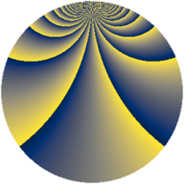# Properties

 Label 570.2.bfLevel $570$ Weight $2$ Character orbit 570.bf Rep. character $\chi_{570}(29,\cdot)$ Character field $\Q(\zeta_{18})$ Dimension $240$ Newform subspaces $1$ Sturm bound $240$ Trace bound $0$

# Related objects

## Defining parameters

 Level: $$N$$ $$=$$ $$570 = 2 \cdot 3 \cdot 5 \cdot 19$$ Weight: $$k$$ $$=$$ $$2$$ Character orbit: $$[\chi]$$ $$=$$ 570.bf (of order $$18$$ and degree $$6$$) Character conductor: $$\operatorname{cond}(\chi)$$ $$=$$ $$285$$ Character field: $$\Q(\zeta_{18})$$ Newform subspaces: $$1$$ Sturm bound: $$240$$ Trace bound: $$0$$

## Dimensions

The following table gives the dimensions of various subspaces of $$M_{2}(570, [\chi])$$.

Total New Old
Modular forms 768 240 528
Cusp forms 672 240 432
Eisenstein series 96 0 96

## Trace form

 $$240q + O(q^{10})$$ $$240q - 30q^{15} + 48q^{19} + 12q^{25} - 48q^{39} + 36q^{45} - 72q^{46} + 216q^{49} - 180q^{51} + 36q^{54} + 60q^{55} + 6q^{60} - 72q^{61} + 120q^{64} - 156q^{66} + 48q^{79} - 120q^{81} - 108q^{84} - 48q^{85} - 36q^{90} - 24q^{91} - 216q^{99} + O(q^{100})$$

## Decomposition of $$S_{2}^{\mathrm{new}}(570, [\chi])$$ into newform subspaces

Label Dim. $$A$$ Field CM Traces $q$-expansion
$$a_2$$ $$a_3$$ $$a_5$$ $$a_7$$
570.2.bf.a $$240$$ $$4.551$$ None $$0$$ $$0$$ $$0$$ $$0$$

## Decomposition of $$S_{2}^{\mathrm{old}}(570, [\chi])$$ into lower level spaces

$$S_{2}^{\mathrm{old}}(570, [\chi]) \cong$$ $$S_{2}^{\mathrm{new}}(285, [\chi])$$$$^{\oplus 2}$$